# Automaton, behaviour of an

(diff) ← Older revision | Latest revision (diff) | Newer revision → (diff)

A mathematical concept describing the interaction of the automaton with its external environment. Thus, for a finite automaton (cf. Automaton, finite) the external environment usually is the set of input words, while its behaviour is the word function generated by the automaton or the event (sometimes the super-event) represented by it. For an automaton over terms (see Automata, algebraic theory of), the set of constant terms is the environment; its behaviour is the class of terms whose values, calculated using the automaton, belong to the isolated subset of elements of the corresponding algebra. For a mosaic structure the environment is the set of initial configurations, while its behaviour consists of the sequences of configurations generated at the various moments of the time set. In general, the concept of the behaviour of (more general) automata is similar to, or is essentially a slight modification of, the concept of the behaviour of finite automata.

The so-called behaviour of an automaton in a random medium is a special case. Here, "medium" is understood to mean a probabilistic automaton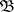, which transforms the output signals of the automatonunder consideration to input signals for. Thus, it may be assumed that the automatonin the random mediumis an autonomous logical network, constructed out of the automataandby connecting the output of each automaton with the input of the other. The behaviour of the automatonin the random mediummay then be regarded as the functioning of this autonomous logical network. The mediumis said to be stationary if it is a single-state automaton. If the output signals of the automatonare regarded as "rewards" or "punishments" for the automaton, the natural problem arises of how to construct an automatonwhose behaviour in the mediumwould be optimal, i.e. would yield the highest possible gain in that medium. It is usually assumed that the output alphabet of the mediumconsists of the letters 0 and 1 and that, as a response to the output signalsof the automaton, the letter 1 is put out, respectively, with probabilities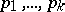. Only the letter 1 is considered to be the "reward" of the automaton.

If the mediumis stationary, the set of states of the autonomous logical network becomes identical with the set of states of the automaton. If, in addition, the output letter of the automatonis unambiguously determined by its state, the functioning of this logical network may be described by a stochastic matrix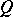of state transitions. The case which is usually considered is that of an ergodic matrix (cf. Ergodicity). Then the function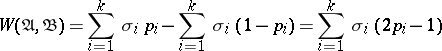is defined, where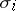is the sum of the final probabilities of all the states which determine the output letter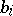. Then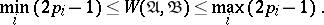If the output signals of the automatondo not depend on the effects of the medium and if they are equally probable, i.e. if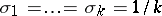, one hasThe function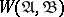is the mathematical expectation of a variable called the gain of the automatonin the medium. One says that the behaviour of the automatonin a mediumis goal-conforming if. The problem of optimal behaviour in a random medium can now be stated as follows. One has to construct a so-called asymptotically optimal sequence of automata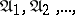such that the mathematical expectation of the gain of automaton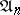tends to the maximum possible gain in this medium, which isasincreases. In the case here considered such a sequence is formed by so-called automata with linear tactics under the condition that. (An automatonwith a linear tactic, with an output alphabet ofletters, has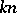states,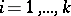,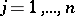, and the following transition functionand output function: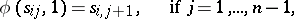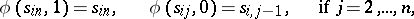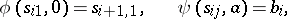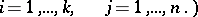The rules governing asymptotically optimal behaviour in a stationary random medium were first studied in mathematical statistics. However, the results obtained in that field can obviously be translated into the language of the theory of automata.

The behaviour of automata is also studied in more complex media, as is the behaviour of collectives of automata in random media. In the latter case the automata are considered to be players, and the rules of the game played by the automata are considered as the medium.

How to Cite This Entry:
Automaton, behaviour of an. Encyclopedia of Mathematics. URL: http://encyclopediaofmath.org/index.php?title=Automaton,_behaviour_of_an&oldid=15570
This article was adapted from an original article by V.B. KudryavtsevYu.I. Yanov (originator), which appeared in Encyclopedia of Mathematics - ISBN 1402006098. See original article